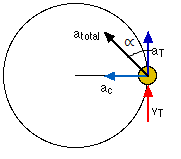Revision Notes for circular motionCircular Motion

Uniform Circular Motion:-Circular motion is said to the uniform if the speed of the particle (along the circular path) remains constant.Circular Motion

Angular Displacement:-

Scalar form:- ?S = r?θ

Angular Velocity:-

Relation between linear velocity (v) and angular velocity (ω):-

Scalar form:- v = rω

AngularAcceleration:-

Relation between linear acceleration (a) and angular acceleration (α):-

Tangential Acceleration and Centripetal AccelerationScalar form:- a= rα

Vector form:-

Here, tangential component,

Relation betweenlinear acceleration (a), angular velocity (ω) and linear velocity (v):-

a=v2/r = ω2/r

Equations of rotational kinematics:-

(a) Angular velocity after a time t second:- ω=ω0+αt

(b) Angular displacement after t second:- θ = ω0t + ½ αt2

(c) Angular velocity after a certain rotation:- ω2 – ω02 = 2αθ

(d) Angle traversed in ‘nth’ second:- θnth = ω0 +α/2 (2n-1)

Time period:- It is the time taken by the particle to complete one rotation.

T= 2π/ω

Frequency:- The number of rotations made by the particle per second is called the frequency of rotation.

If f is the frequency, the particle describes 2πf radians per second.

ω = 2πf

So, f = 1/T

Centripetal force:- The force, acting along the radius towards the center, which is essential to keep the body moving in a circle with uniform speed is called centripetal force. It acts always along the radius towards the center. A centripetal force does no work.

F = mv2/r = mrω2

Centrifugal force:- Centrifugal force is the fictitious force which acts on a body, rotating with uniform velocity in a circle, along the radius away from the center. Magnitude of centrifugal force is,

F = mv2/r

Centripetal and centrifugal forces are equal in magnitude and opposite in direction. They cannot be termed as action and reaction since action and reaction never act on same body.

Road offering no frictional resistance, θ = tan-1 (v2/rg)

Road offering frictional resistance, vmax = √rg(µ+tanθ/1-µtanθ)

Bending of Cyclist:- θ = tan-1 (v2/rg)

(a) Velocity of the cyclist:- Greater the velocity, greater is his angle of inclination with the vertical.

(b) Radius of curvature:- Smaller the radius, greater is the angle with the vertical.

Time period of conical pendulum:-T = 2π √lcosθ/g

Motion in a vertical circle/looping the loop:-

The minimum velocity of the body, at the lowest point, required to take the body round a vertical circle is √5gr.

So, v = √5gr

The minimum tension in the string, at the lowest point, required to take the body around the vertical circle is equal to six times the weight of the body.

So, T1= 6 mg

(a) For lowest point A and highest point B, TA – TB = 6 mg

VA2 = VB2+4gl

VA≥√5gl

VB≥√gl

(b) Condition for oscillation:- VA?√2gl

(c) Condition for leaving circular path:- √2gl< VA?√5gl

Non-uniform circular motion:-

(a) The velocity changes both in magnitude as well as in direction.

(b) The velocity vector is always tangential to the path.

(c) The acceleration vector is not perpendicular to the velocity vector.

(d) The acceleration vector has two components.

(i) Tangential acceleration at changes the magnitude of velocity vector and is defined as, at = dv/dt

(ii) Normal acceleration or centripetal acceleration ac changes the direction of the velocity vector and is defined as,ac = v2/r

(iii) The total acceleration is the vector sum of the tangential and centripetal acceleration.

So, a = √at2+ac2